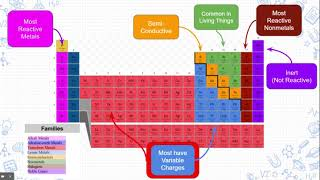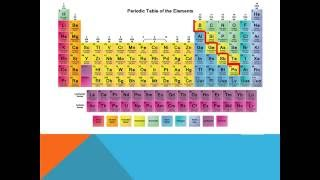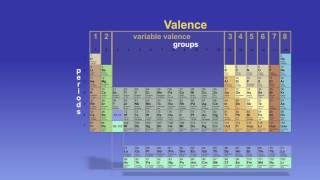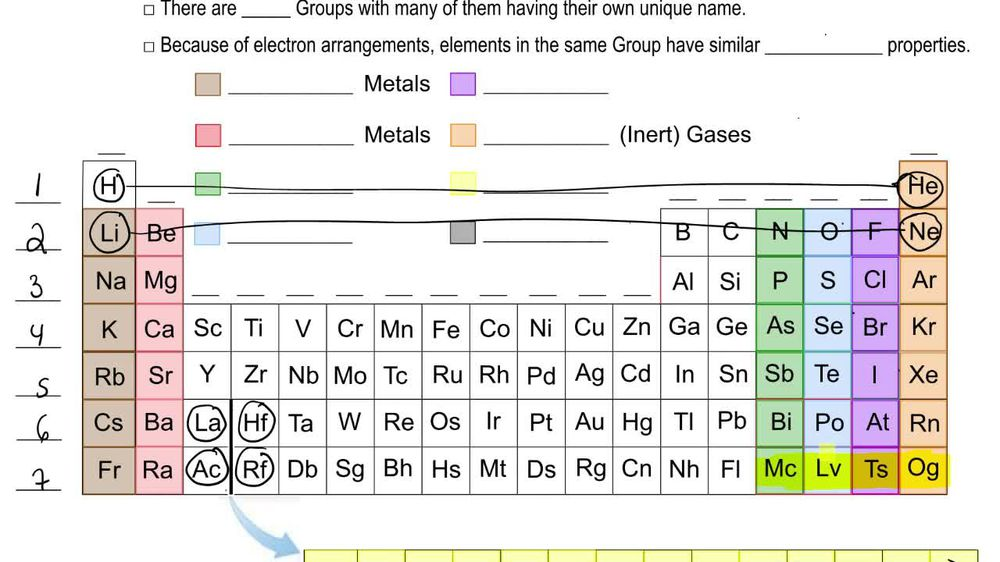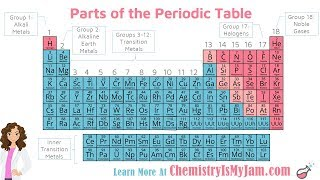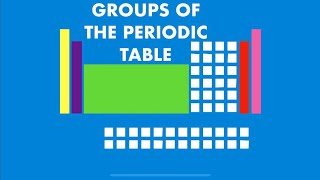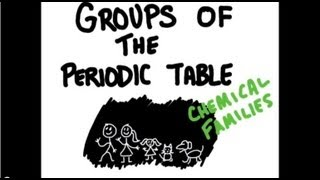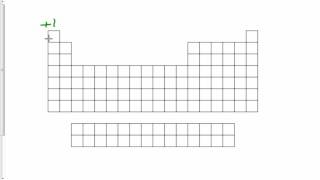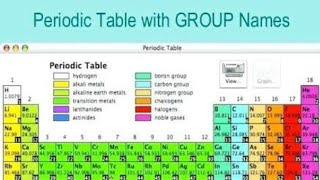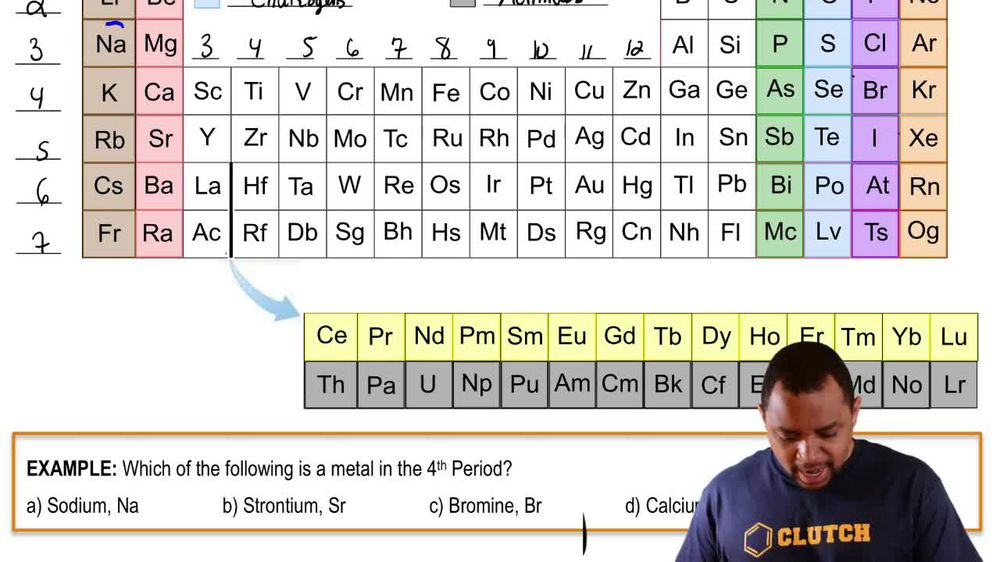Start typing, then use the up and down arrows to select an option from the list.
1. 2. Atoms & Elements2. Periodic Table: Group Names
Problem

# (b) Without looking at a periodic table, list as many alkaline earth metals as you can. (There are five common ones.)

Relevant Solution1m
Play a video:
Hello everyone today. We are the following question without referring to the periodic table. Which are the following are examples of alkaline earth metals. So alkaline earth metals are going to be in Group two A. And Group two A elements are going to be such examples of beryllium, there's magnesium, there's calcium, strontium, bury him and radium. So let's go through these answer choices and see which ones contain these elements. So answer choice A. Has magnesium, barium And cesium. However, Cesium is not a part of a group two a elements. And so that's not going to be the answer B. Has sodium. That's automatically not going to be a group to element. So its choice B. Can be erased as well as answer choices. D. And so if you look at sea there's calcium which we have, there's strontium which we have and there's barium which we have. This makes anti choice C. Correct. And with that we've answered the question overall. I hope this helped. And until next time.